Test: Amplitude Modulation

# Test: Amplitude Modulation

Test Description

## 20 Questions MCQ Test GATE ECE (Electronics) 2023 Mock Test Series | Test: Amplitude Modulation

Test: Amplitude Modulation for Electronics and Communication Engineering (ECE) 2023 is part of GATE ECE (Electronics) 2023 Mock Test Series preparation. The Test: Amplitude Modulation questions and answers have been prepared according to the Electronics and Communication Engineering (ECE) exam syllabus.The Test: Amplitude Modulation MCQs are made for Electronics and Communication Engineering (ECE) 2023 Exam. Find important definitions, questions, notes, meanings, examples, exercises, MCQs and online tests for Test: Amplitude Modulation below.
Solutions of Test: Amplitude Modulation questions in English are available as part of our GATE ECE (Electronics) 2023 Mock Test Series for Electronics and Communication Engineering (ECE) & Test: Amplitude Modulation solutions in Hindi for GATE ECE (Electronics) 2023 Mock Test Series course. Download more important topics, notes, lectures and mock test series for Electronics and Communication Engineering (ECE) Exam by signing up for free. Attempt Test: Amplitude Modulation | 20 questions in 60 minutes | Mock test for Electronics and Communication Engineering (ECE) preparation | Free important questions MCQ to study GATE ECE (Electronics) 2023 Mock Test Series for Electronics and Communication Engineering (ECE) Exam | Download free PDF with solutions
 1 Crore+ students have signed up on EduRev. Have you?
Test: Amplitude Modulation - Question 1

### A carrier is simultaneously modulated by two sine waves with modulation indices of 0.4 and 0.3. The resultant modulation index will be

Detailed Solution for Test: Amplitude Modulation - Question 1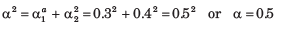Test: Amplitude Modulation - Question 2

### In a DSB-SC system with 100% modulation, the power saving is

Detailed Solution for Test: Amplitude Modulation - Question 2

In previous solution Pc = 2/3P. If carrier is suppressed then 2/3 P or 66% power will be saved.

Test: Amplitude Modulation - Question 3

### A 10 kW carrier is sinusoidally modulated by two carriers corresponding to a modulation index of 30% and 40% respectively. The total radiated power(is

Detailed Solution for Test: Amplitude Modulation - Question 3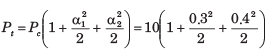= 11.25 kW

Test: Amplitude Modulation - Question 4

In amplitude modulation, the modulation envelope has a peak value which is double the unmodulated carrier value. What is the value of the modulation index ?

Detailed Solution for Test: Amplitude Modulation - Question 4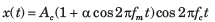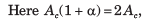Thus α = 1, therefor modulation index is 1 or 100% modulation.

Test: Amplitude Modulation - Question 5

If the modulation index of an AM wave is changed from 0 to 1, the transmitted power

Detailed Solution for Test: Amplitude Modulation - Question 5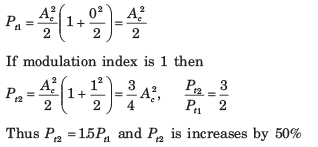Test: Amplitude Modulation - Question 6

A diode detector has a load of 1 kΩ shunted by a 10000 pF capacitor. The diode has a forward resistance of 1 Ω. The maximum permissible depth of modulation,so as to avoid diagonal clipping, with modulating signal frequency fo 10 kHz will be

Detailed Solution for Test: Amplitude Modulation - Question 6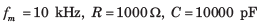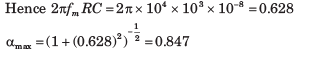Test: Amplitude Modulation - Question 7

An AM signal is detected using an envelop detector.The carrier frequency and modulating signal frequency are 1 MHz and 2 kHz respectively. An appropriate value for the time constant of the envelope detector is.

Detailed Solution for Test: Amplitude Modulation - Question 7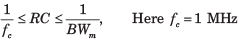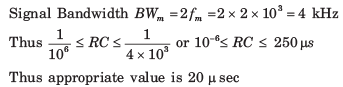Test: Amplitude Modulation - Question 8

A 1 MHz sinusoidal carrier is amplitude modulated by a symmetrical square wave of period 100 μ sec. Which of the following frequencies will NOT be present in the modulated signal?

Detailed Solution for Test: Amplitude Modulation - Question 8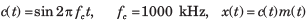Expressing square wave as modulating signal m(t)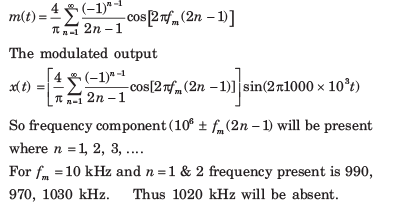Test: Amplitude Modulation - Question 9

For an AM signal, the bandwidth is 10 kHz and the highest frequency component present is 705 kHz. The carrier frequency used for this AM signal is

Detailed Solution for Test: Amplitude Modulation - Question 9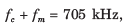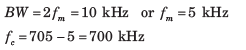Test: Amplitude Modulation - Question 10

A message signal m(t) = sinct + sinc2(t)  modulates the carrier signal (t) = Acos2πfct . The bandwidth of the modulated signal is

Detailed Solution for Test: Amplitude Modulation - Question 10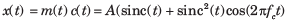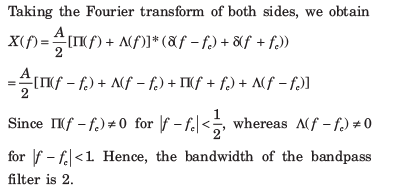Test: Amplitude Modulation - Question 11

Consider the system shown in figure . The average value of m(t) is zero and maximum value of
|m(t)| is M. The square-law device is defined by y(t) = 4x(t) +10 x(t).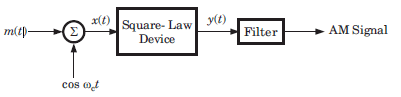Que: The value of M, required to produce modulation index of 0.8, is

Detailed Solution for Test: Amplitude Modulation - Question 11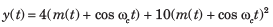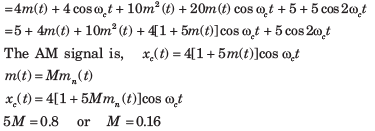Test: Amplitude Modulation - Question 12

Consider the system shown in figure . The average value of m(t) is zero and maximum value of
|m(t)| is M. The square-law device is defined by y(t) = 4x(t) +10 x(t).Let W be the bandwidth of message signal m(t). AM signal would be recovered if

Detailed Solution for Test: Amplitude Modulation - Question 12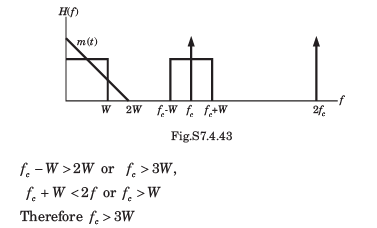Test: Amplitude Modulation - Question 13

A super heterodyne receiver is designed to receive transmitted signals between 5 and 10 MHz. High-side tuning is to be used. The tuning range of the local oscillator for IF frequency 500 kHz would be

Detailed Solution for Test: Amplitude Modulation - Question 13

Since High-side tuning is used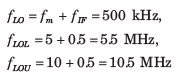Test: Amplitude Modulation - Question 14

A super heterodyne receiver uses an IF frequency of 455 kHz. The receiver is tuned to a transmitter having a carrier frequency of 2400 kHz. High-side tuning is to be used. The image frequency will be

Detailed Solution for Test: Amplitude Modulation - Question 14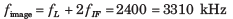Test: Amplitude Modulation - Question 15

In the circuit shown in fig, the transformers are center tapped and the diodes are connected as shown in a bridge. Between the terminals 1 and 2 an a.c. voltage source of frequency 400 Hz is connected. Another a.c. voltage of 1.0 MHz is connected between 3 and 4. The output between 5 and 6 contains components at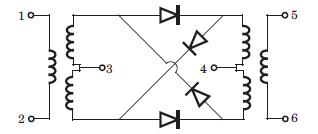Detailed Solution for Test: Amplitude Modulation - Question 15

The given circuit is a ring modulator. The output is DSB-SC signal. So it will contain m(t) cos( nwct) where n = 1,2, 3...... Therefore there will be only (1 MHz +- 400 Hz) frequency component.

Test: Amplitude Modulation - Question 16

A superheterodyne receiver is to operate in the frequency range 550 kHz-1650 kHz, with the intermediate frequency of 450 kHz. Let R = cmax / cmin   denote the required capacitance ratio of the local oscillator and I denote the image frequency (in kHz) of the incoming signal. If the receiver is tuned to 700 kHz, then

Detailed Solution for Test: Amplitude Modulation - Question 16

fmax = 1650 + 450 = 2100 kHz

fmin = 550 + 450 = 1000 kHz.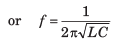Test: Amplitude Modulation - Question 17

Consider a system shown in Figure . Let X( f ) and Y( f ) denote the Fourier transforms of x(t) and y(t) respectively. The ideal HPF has the cutoff frequency 10 kHz. The positive frequencies where Y( f ) has spectral peaks are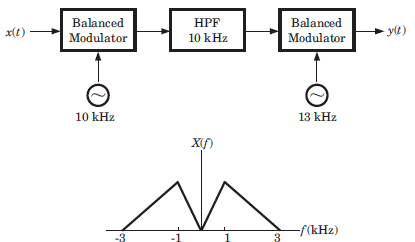Detailed Solution for Test: Amplitude Modulation - Question 17

Since X (f) has spectral peak at 1 kHz so at the output of first modulator spectral peak will be at (10 + 1) kHz and (10  1) kHz. After passing the HPF frequency component of 11 kHz will remain. The output of 2nd modulator will be (13 +11) kHz. So Y (f) has spectral peak at 2 kHz and 24 kHz.

Test: Amplitude Modulation - Question 18

In fig.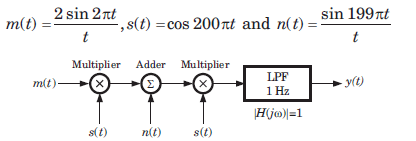The output y(t) will be

Detailed Solution for Test: Amplitude Modulation - Question 18

m(t).s(t) = y1(t)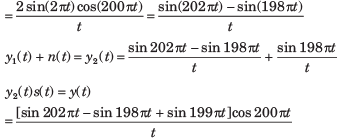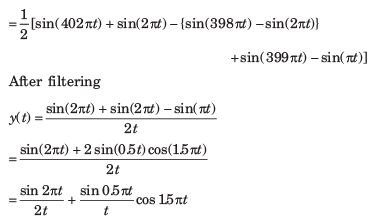Test: Amplitude Modulation - Question 19

What is the carrier frequency in an AM wave when its highest frequency component is 850Hz and the bandwidth of the signal is 50Hz?

Detailed Solution for Test: Amplitude Modulation - Question 19

Upper frequency = 850Hz
Bandwidth = 50Hz
Therefore lower Frequency = 850 - 50= 800 Hz
Carrier Frequency = (850-800)/2
= 825 Hz

Test: Amplitude Modulation - Question 20

Suppose we wish to transmit the signal x(t) = sin 200πt + 2 sin 400πt using a modulation that create the signal g(t) = x(t) sin 400πt. If the product g(t) sin 400πt is passed through an ideal LPF with cutoff frequency 400π and pass band gain of 2, the signal obtained at the output of the LPF is

Detailed Solution for Test: Amplitude Modulation - Question 20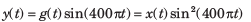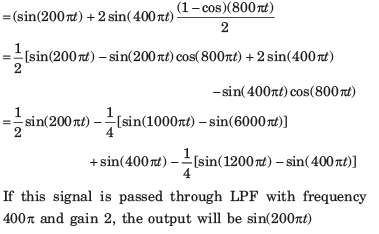## GATE ECE (Electronics) 2023 Mock Test Series

21 docs|263 tests
 Use Code STAYHOME200 and get INR 200 additional OFF Use Coupon Code
Information about Test: Amplitude Modulation Page
In this test you can find the Exam questions for Test: Amplitude Modulation solved & explained in the simplest way possible. Besides giving Questions and answers for Test: Amplitude Modulation , EduRev gives you an ample number of Online tests for practice

## GATE ECE (Electronics) 2023 Mock Test Series

21 docs|263 tests